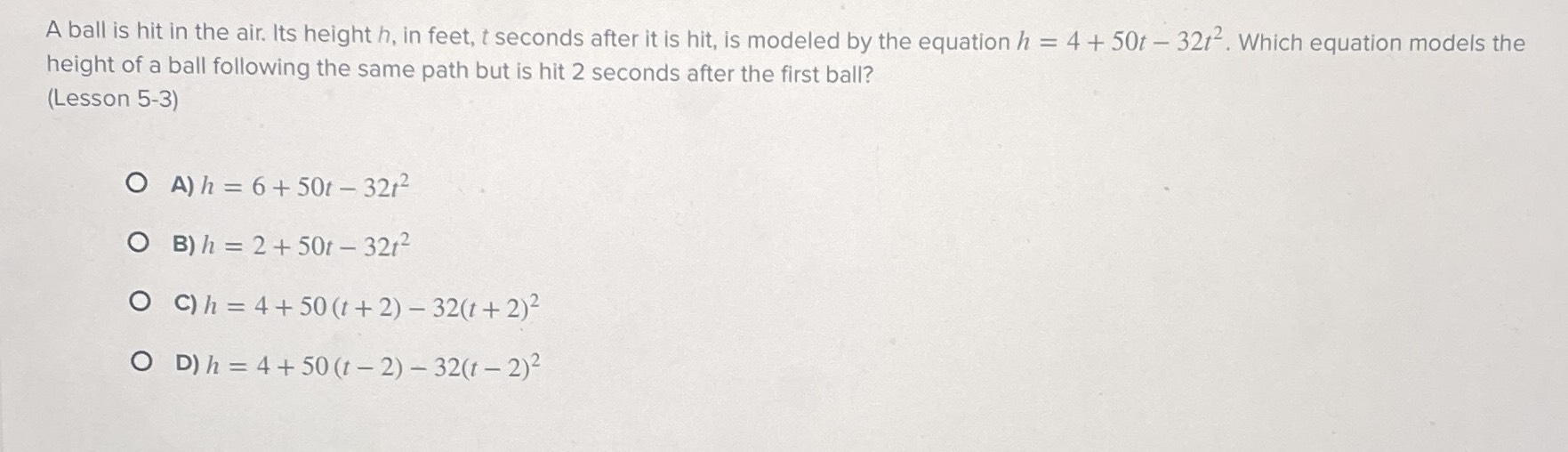### ¿Todavía tienes preguntas de matemáticas?

Pregunte a nuestros tutores expertos
Algebra
PreguntaA ball is hit in the air. Its height $$h$$ , in feet, $$t$$ seconds after it is hit, is modeled by the equation $$h = 4 + 50 t - 32 t ^ { 2 }$$ . Which equation models the height of a ball following the same path but is hit $$2$$ seconds after the first ball?

A) $$h = 6 + 50 t - 32 t ^ { 2 }$$

B) $$h = 2 + 50 t - 32 t ^ { 2 }$$

C) $$h = 4 + 50 ( t + 2 ) - 32 ( t + 2 ) ^ { 2 }$$

D) $$h = 4 + 50 ( t - 2 ) - 32 ( t - 2 ) ^ { 2 }$$